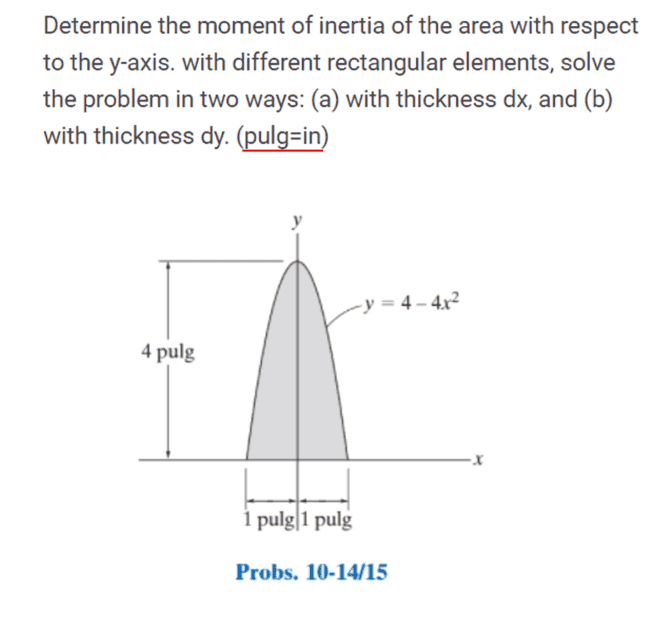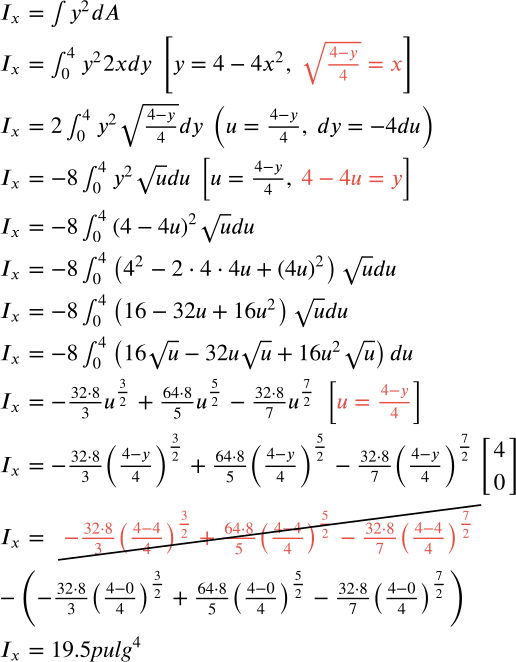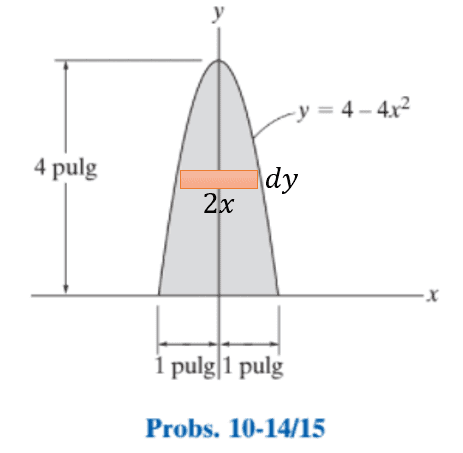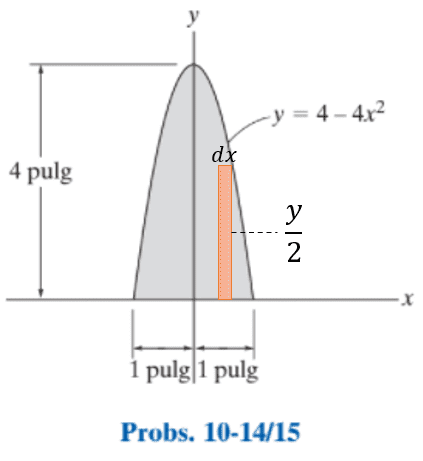# How to determine the same moment of inertia in two different ways?

Tapias5000
Homework Statement:
determine the moment of inertia of the area with respect to the y-axis. with different rectangular elements, solve the problem in two ways: (a) with thickness dx, and (b) with thickness dy.
Relevant Equations:
## I_x=\int _{ }^{ }y^2dA ##My solution isnow I am asked for the same result but in this form but I don't know where to start.Gold Member
$$2\sigma \int_0^1 x^2 y \ \ dx=32\sigma \int_0^{\pi/2} \sin^2\theta \cos^2\theta d\theta$$
where ##\sigma## is area mass density of board.

Last edited:
Tapias5000
$$2\sigma \int_0^1 x^2 y \ \ dx=32\sigma \int_0^{\pi/2} \sin^2\theta \cos^2\theta d\theta$$
where ##\sigma## is area mass density of board.
waat, Can you tell me the name of this method?

Gold Member
I misinterpreted y so
$$2\sigma \int_0^1 x^2 y \ \ dx=2\int_0^1 x^2 (4-4x^2) \ \ dx$$
This a way (b).
Homework Statement:: determine the moment of inertia of the area with respect to the y-axis. with different rectangular elements, solve the problem in two ways: (a) with thickness dx, and (b) with thickness dy.
Relevant Equations:: ## I_x=\int _{ }^{ }y^2dA ##
This relevant equation seems inappropriate because x should be squared for y-axis rotation inertia.
For (a)

$$2\sigma\int_0^4 dy \int_0^{\frac{\sqrt{4-y}}{2}} x^2 dx$$

Last edited: